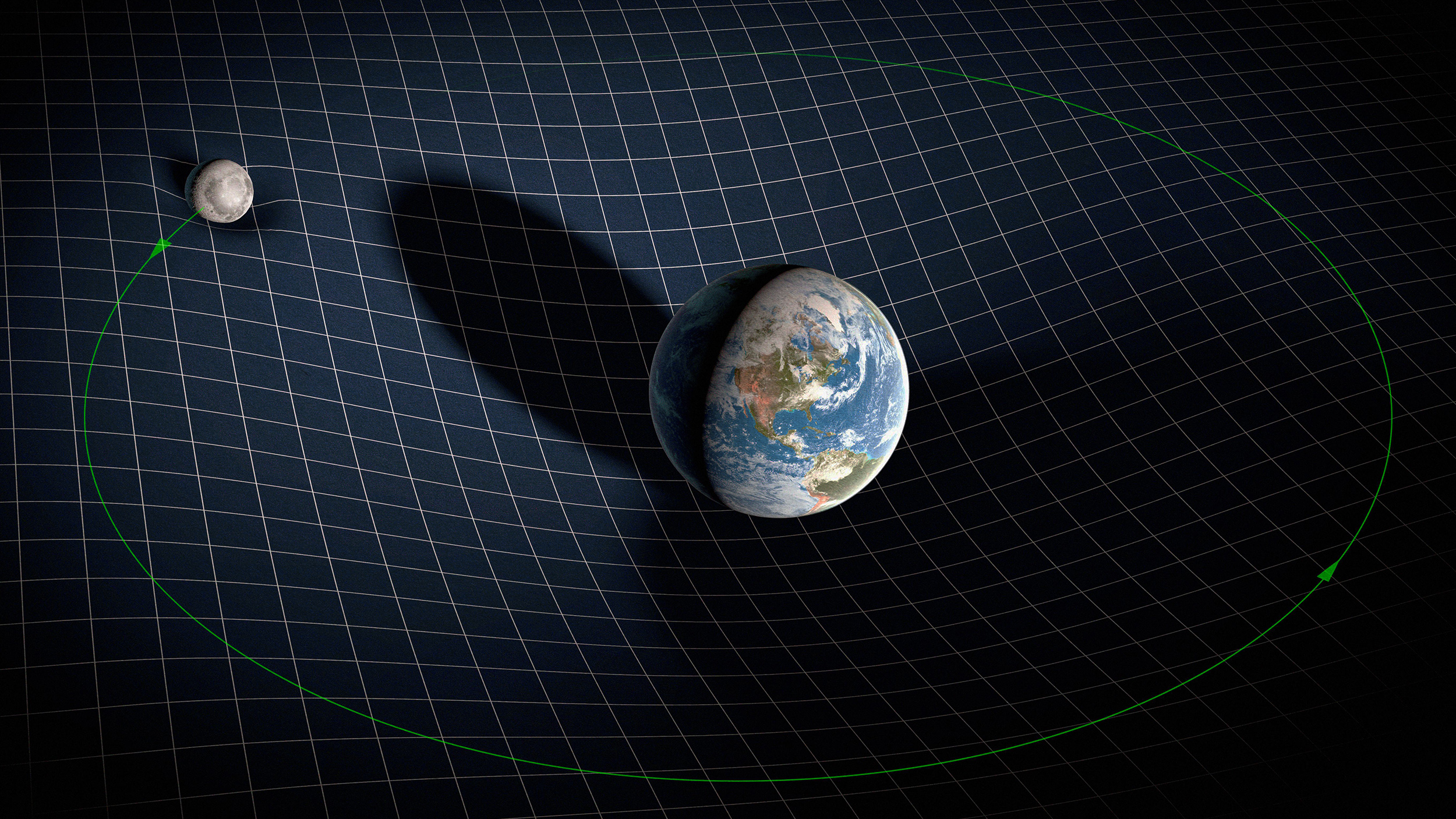Hard Science

# How the Moon is helping us confirm Einstein’s relativity

This measurement is crucial to confirm that one of the assumptions of Einstein’s theory of gravity is valid.The gravitational fields of the Earth and Moon distorting the fabric of space-time. / Science Photo Library / Alamy
Key Takeaways
• Mass can be thought of as how much “stuff” an object is made of. It is what causes the gravitational force between two objects, and it is what makes objects difficult to move.
• In introductory physics classes and even in Einstein’s most advanced theory, the three "kinds" of mass are thought to be one and the same. But there is no fundamental reason why they must be.
• A series of precise measurements of the Moon confirms that two of these "kinds" of mass are the same, which also helps validate Einstein's theory of general relativity.

It is often possible to use data gathered for one purpose to study something else. For example, a recent paper used half a century’s worth of observations of the Moon’s orbit to perform subtle and precise tests of the nature of gravity, as well as some of the key assumptions that went into deriving Einstein’s theory of general relativity.

## One mass, three functions

Mass can be thought of as how much “stuff” an object is made of. It is what causes the gravitational force between two objects, and it is what makes objects difficult to move. Essentially, it is serving three different functions. First, mass generates a gravitational field that will exert a force on other objects, so we could call this “active gravitational mass.” Second, mass can feel the gravitational effects from surrounding objects, and we could call this “passive gravitational mass.” Third, mass resists changes in motion — which is why pushing a big rock is difficult — so we might call this “inertial mass.”

In introductory physics classes and even in Einstein’s most advanced theory, the three “kinds” of mass are thought to be one and the same. But there is no fundamental reason why they must be. It could be that each one is different. Given the idea that they are the same is a key assumption of Einstein’s theory of gravity, it is imperative that we test the conjecture.

So that’s what researchers have done. On July 21, 1969, the Apollo 11 astronauts put a laser reflector on the surface of the Moon, followed by additional reflectors on subsequent lunar missions. Since then, researchers have been able to monitor the distance between the Earth and the Moon. This is an incredibly precise measurement, accurate to within a millimeter, which would be like measuring the distance between New York and Los Angeles with a precision equivalent to the width of a human hair. One outcome was that scientists have determined that the Moon is moving away from the Earth at the stately pace of 1.5 inches (3.8 cm) per year.

## Active vs. passive gravitational mass

The most recent paper investigated whether active mass and passive mass are the same. To do so, researchers exploited a geological feature of the Moon. The Apollo missions determined that the maria (the big dark spots on the face of the moon) were rich in iron, while the highlands of the moon were rich in aluminum. Because the maria were formed from lava coming from the interior of the Moon, the researchers assumed that the Moon’s mantle was iron-rich. The highlands, being the outer part of the Moon, should reflect the chemical makeup of the aluminum-rich lunar crust.

In the case where active and passive gravitational mass are the same, the gravitational tug of the iron on the aluminum should be the same as the aluminum on the iron. However, if active and passive gravitational mass are different, there should be a net force. This net force would be similar to the tides on Earth and would cause the Moon’s orbit to speed up or slow down.

Using over half a century of measurements of the location of the Moon (from April 1970 to April 2022), astronomers were able to determine that the orbit of the Moon was slowing by the tiny amount of 25.8 arcseconds per century. At this rate, it would take over 14,000 years for the Moon to fall a single degree behind where it would be if it continued to travel at the speed at which it is moving today.

From this precise measurement, researchers concluded that the active and passive forms of mass were essentially identical. If they are different, the difference is less than one part in 26 trillion.

## Confirming Einstein

This measurement is crucial to confirm that one of the assumptions of Einstein’s theory of gravity is valid. Were it not, it could bring into question many facets of modern astronomy, including dark matter and dark energy. However, the recent measurement validates our current theoretical framework. Once again, Einstein is shown to be correct.

Related
Newton thought that gravitation would happen instantly, propagating at infinite speeds. Einstein showed otherwise; gravity isn't instant.
We have two descriptions of the Universe that work perfectly well: General Relativity and quantum physics. Too bad they don't work together.
How are we to deal with the quantization of spacetime and gravity?
The theory is accurate within at least one part in a quadrillion.
Though a single measurement is not enough to definitively decide the debate, this is a major win for dark matter proponents.

Up Next
Because the milk was thin and had an unnatural, bluish tint, vendors stirred in additives such as chalk, flour, eggs, and Plaster-of-Paris.